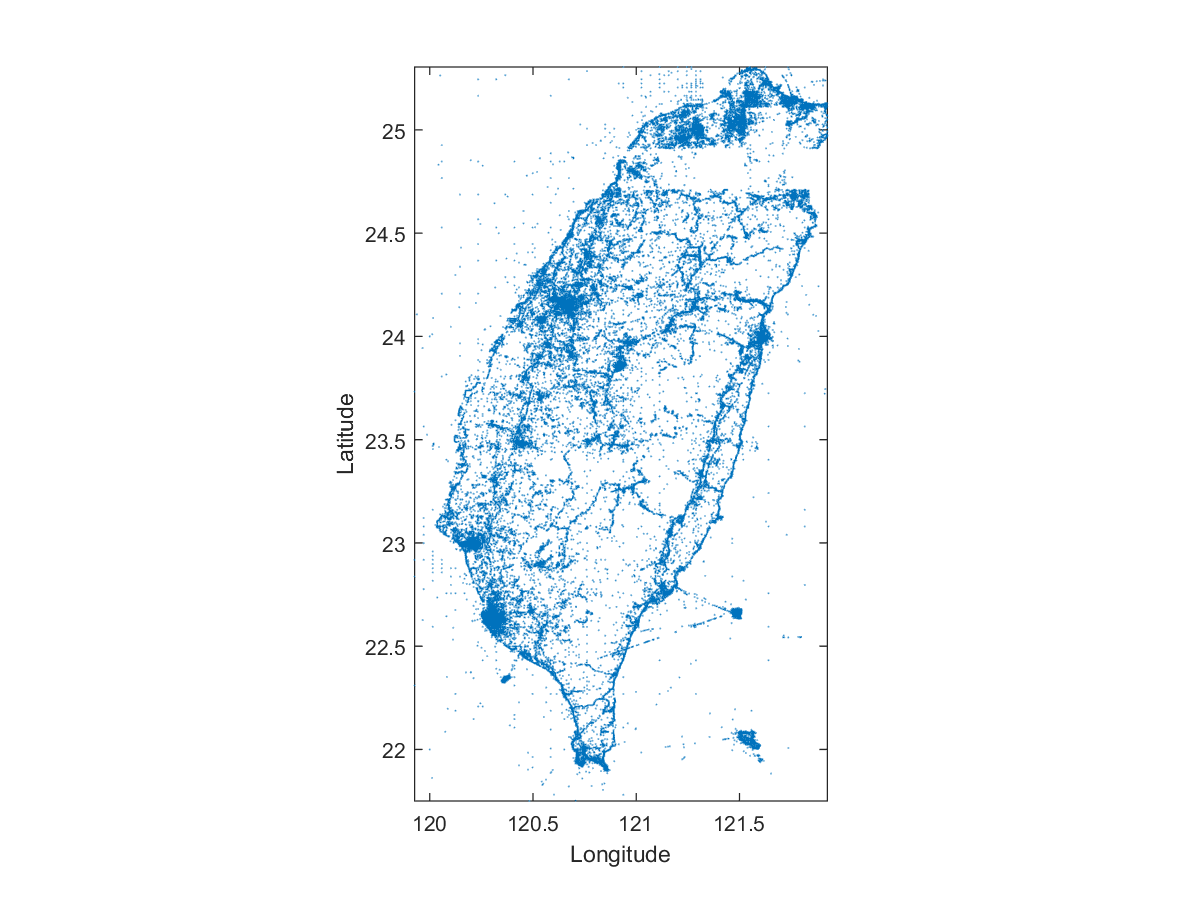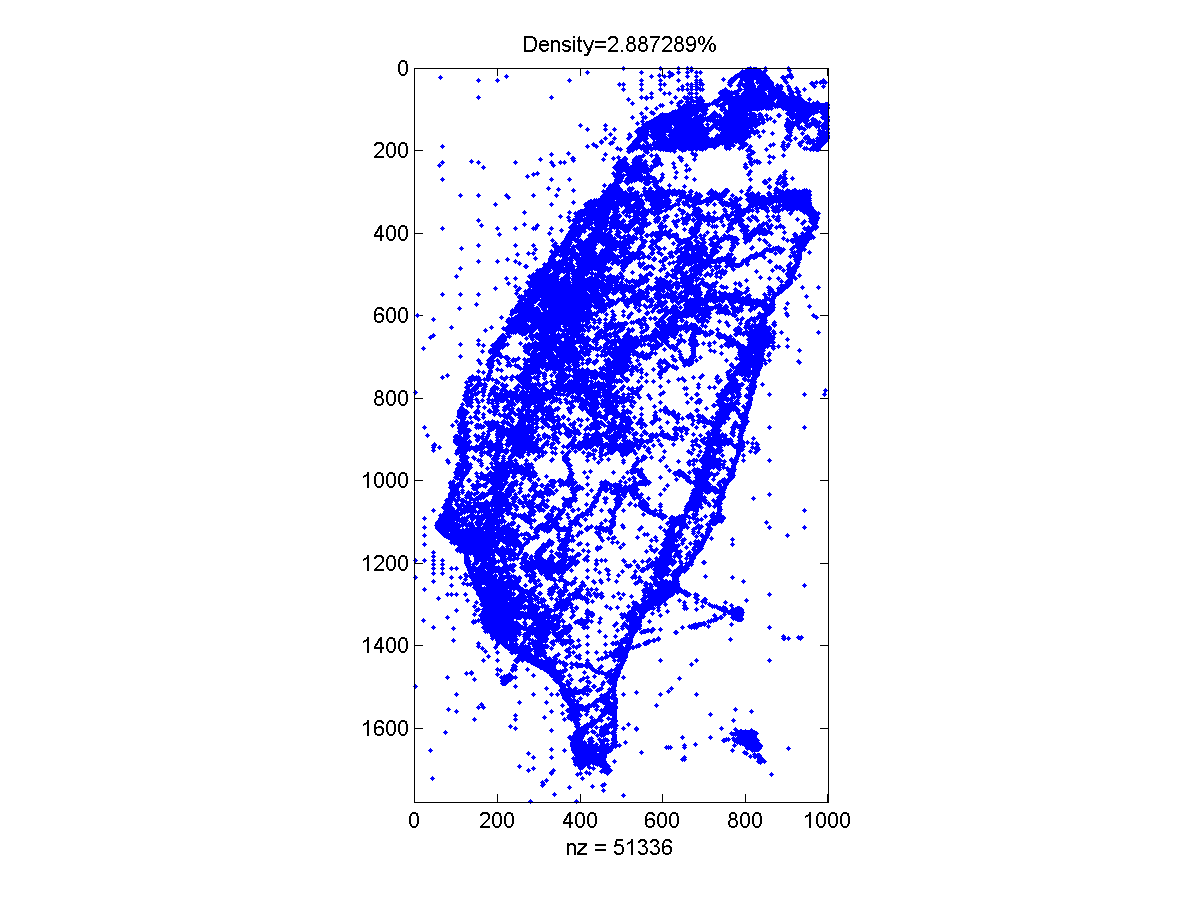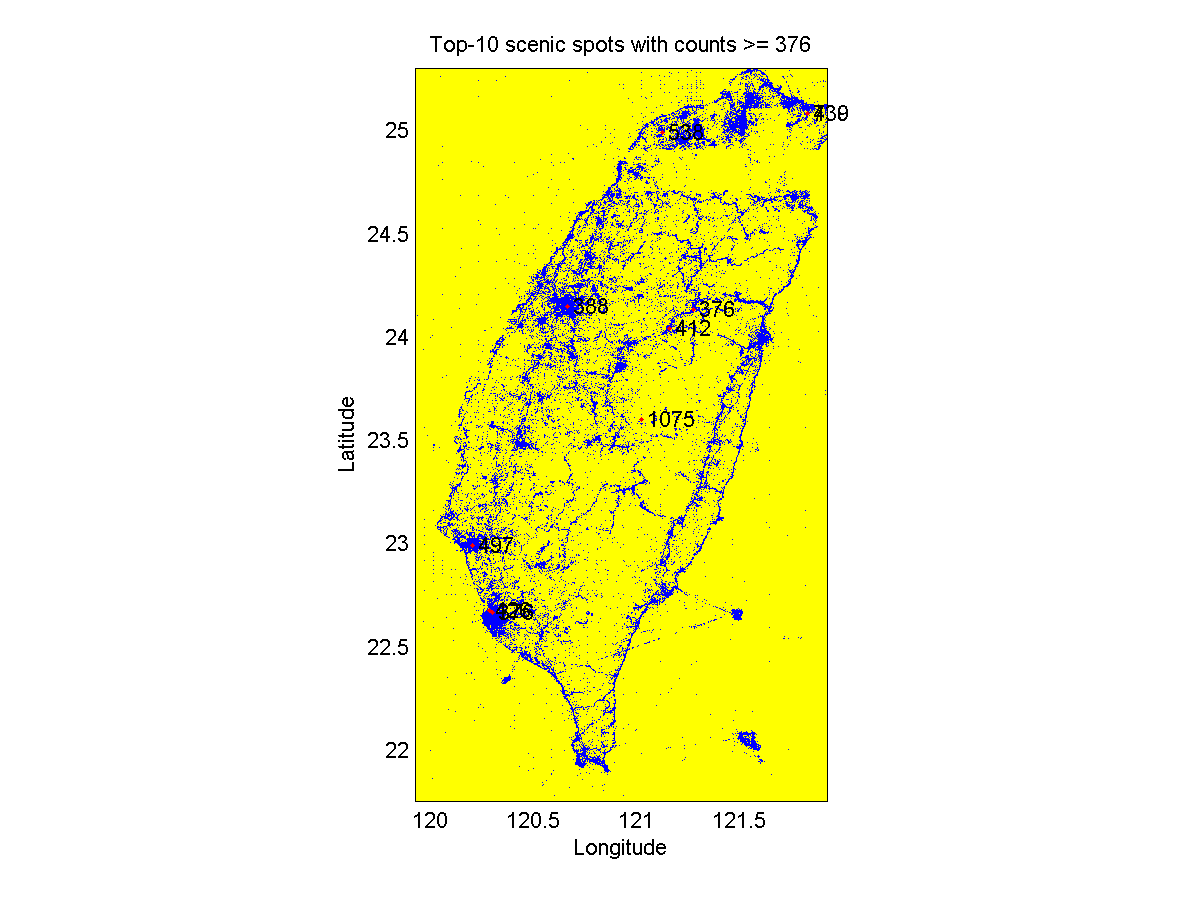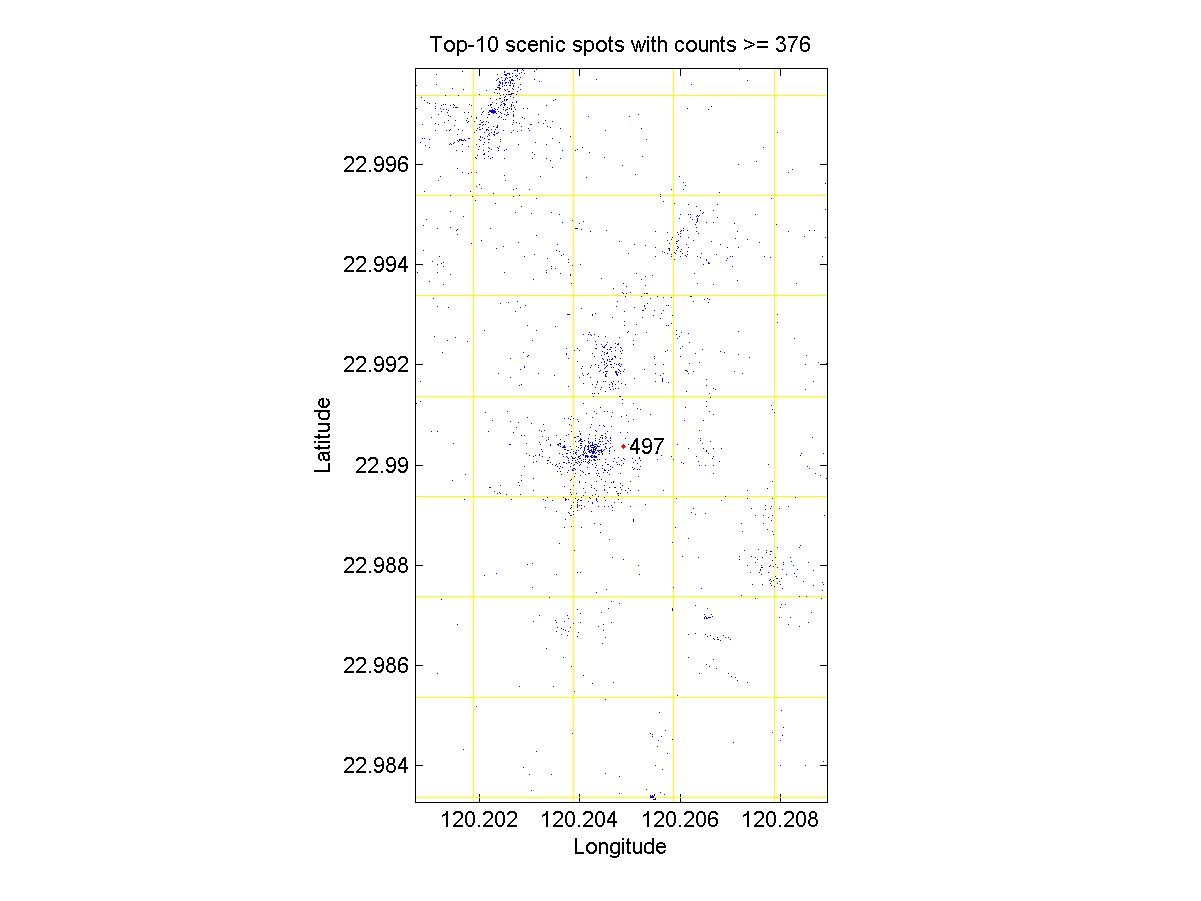## Part 1

1. 請解釋為何需要使用稀疏矩陣？
Ans: 若一個矩陣中的大部分元素為零，則此矩陣為稀疏矩陣，若採取一般矩陣的儲存方式，則其使用的記憶體空間和矩陣總元素個數成正比，造成記憶體空間的浪費，因此我們必須採用稀疏矩陣的陣列儲存方式，才能利用較少的記憶體空間來儲存這一類稀疏矩陣。
2. 請使用 MATLAB 的 sparse 指令，產生一個 5x10 稀疏矩陣 S，包含以下元素： (1,4)=5 (5,1)=1 (4,3)=7
Ans: S=sparse([1 5 4],[4 1 3], [5 1 7],5,10);
3. 假設有一個 100X100 的矩陣 A，其元素大部分是零，只有下列幾個特定元素不為零： A(1, 3)=4 A(2, 5)=10 A(35, 70)=4 A(54, 98)=5
1. 請用 sparse 指令來建立此稀疏矩陣 A。
2. A 佔掉多少位元組？
3. 若 A 是完全矩陣，且是 double 的資料型態，則會佔去多少位元組？
4. 若 A 是完全矩陣，且是 uint8 的資料型態，則會佔去多少位元組？
5. 使用 spy 來畫出非零元素的分佈狀況。
4. 一個 10x10 的矩陣，資料型態為 double，請問當非零元素大於何值時，將此矩陣以稀疏矩陣來存放並無法達到節省記憶體之目的？（假設我們使用 MATLAB 第七版。）
Hint: 16*nnz + 8*n + 8 > 8*m*n
5. 稀疏矩陣只存非零元素是為了節省記憶體空間。請分別計算 8x4 及 4x8 的 double 矩陣中，能讓稀疏矩陣達到目的之非零元素的最大個數。（假設我們使用 MATLAB 第七版。）
答案：13, 11
6. 若一個 10x10 矩陣 A 的行列式值不為 0，請問：
1. 此矩陣的最小密度為？（矩陣密度可定義為非零元素所佔的百分比。）
2. 若此矩陣資料以 double 存放，試問其 A 所佔的空間是 B = sparse(A) 的多少倍？
7. 給定一個 MATLAB 工作空間中的稀疏矩陣，假設我們將此矩陣中的某一個非零元素改成零，此時 MATLAB 會立刻釋放記憶體空間嗎？（也就是說，此矩陣所佔用的空間會立刻變小嗎？）為什麼要這樣設計？
8. 若 nodes = [0 1 1 2 2 ]，請問 treeplot(nodes) 會畫出的樹狀圖為何？
Ans: o / \ o \ / \ \ o o o
9. 貧窮的小明想用 eig 指令對一個紀錄五千個節點的連線矩陣（其大小為 5000x5000）求得特徵值，卻發現記憶體不足，在無法升級記憶體的情況下，他該如何是好呢？
Ans: 先將矩陣改用稀疏矩陣的型態進行儲存，再用eigs()對矩陣求特徵值即可。

## Part 2

1. Scenic spot identification: In this exercise, you are going to deal with a real-world problem of extracting scenic spots from the latitude & longitude infomation from the photos archives such as flickr. You can download the data file at here, which contains three columns of longitudes, latitude, and URL of the photos. You can plot the data distribution as follows:

Example 1: 05-稀疏矩陣/goShowPhotoLocation.mfid=fopen('photoRawData276299.txt'); contents = textscan(fid, '%f %f %s'); fclose(fid); x=contents{1}; y=contents{2}; h=plot(x, y, '.'); axis image xlabel('Longitude'); ylabel('Latitude'); set(h, 'markersize', 1); print('-dpng', mfilename);From the above plot, you can almost see the shape of Taiwan. Probably you can also identify several big cities. Our goal is to identify the mostly visited places of Taiwan, or scenic spots. One way to achieve the goal is to partition the whole map into grids and counting the photos in each square. The square with the higher counts will be regarded as the scenic spots. To do so along the x-axis, please following the general steps as follows:
1. Find the center points of each square along the x-axis:
xCenter=linspace(min(x), max(x), m);
Note that m is used to determine the size of each square.
2. Find the boundary of each square along the x-axis:
xBoundary=(xCenter(1:end-1)+xCenter(2:end))/2;
3. Determine the intervl of of each photo's location along the x-axis. For instance, if you want to determine the interval indices of photos 3 to 5 when m=1000, use the command:
squareIndex=rangeSearchBin(x(3:5), xBoundary);
The result is [278; 199; 960]. (Note that rangeSearchBin is available within the Utility Toolbox.)
Please follow the above procedure to find the counts along the y-axis, and then combine them to get the counts for all the squares. When m (resolution on the x-axis) is 1000, n (resolution on the y-axis) is around 1778, so you should initialize a sparse matrix countMat of size m by n to store all the count information. This is just like a 2d histogram.) You need to complete the following tasks, assuming m = 1000.
1. Plot the sparse matrix countMat using spy command as shown next. What is the density of the sparse matrix?2. Plot the grid lines and the data distribution, and overlay the top-10 scenic spots with their counts, as follows:Note that you should be able to zoom in to view the details. For instance, you can zoom in the scenic spot at Tainan to get the following plot:3. Please find a way to specify a region as the scenic spot instead of a single dot. Here you can use convhull to find the region and then plot it on top of the data distribution.
When m is 1000, the size of the square is about 200000/m = 200 meters. This indicates we are interested in big-area scenic spots, such as Taipei 101 and its surroundings. When m is 10000, the size of the square is about 200000/m = 20 meters. This indicates we are interested in small-area scenic spots, possibly some famous restaurant (for example, 鼎泰豐). Please repeat the above process when m is 10000.
2. Improvement to scenic spot identification: Apparently the method proposed in the previous exercise for scenic spot identification is not quite satisfactory since some scenic spots are likely to be divided by a grid line. Please propose your own methods and demonstrate the improved results.
3. Tree representation: Plot a tree specified by the vector [0 1 1 2 2 1 6 6 6 7 7 11].

MATLAB程式設計：進階篇